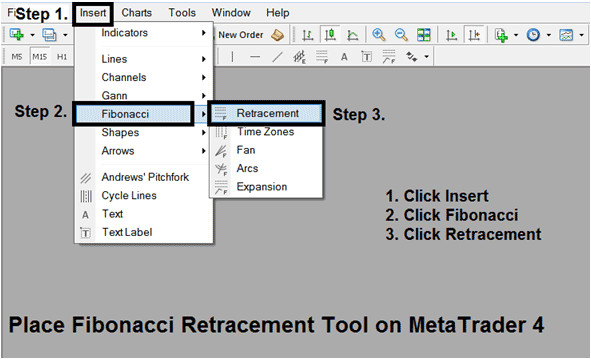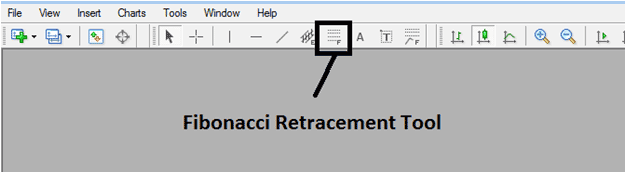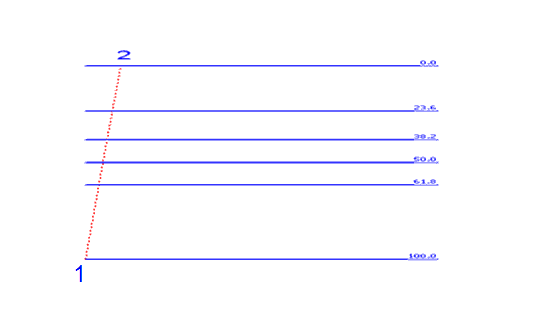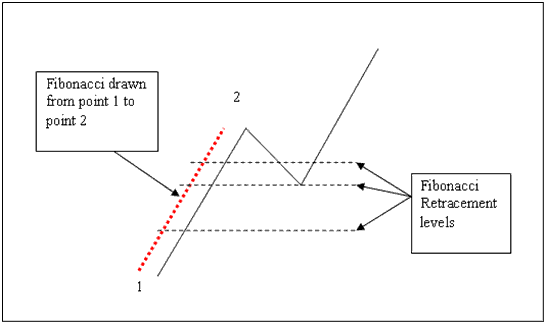# How to Draw Fibonacci Retracement Levels on MetaTrader 4 Platform

To calculate these price pullbacks on the Gold price chart for example the MetaTrader 4 platform we use Fibonacci retracement levels tool/indicator as shown on the image below.

If you want to analyze some Gold price charts using this tool, it is already provided for in the MT4 platform, the following procedure is used to add this tool on the MetaTrader 4 Software. On The MT4 this Fibonacci retracement tool will automatically draw the retracement levels on a price chart once this indicator is placed on the Gold price chart.

To add this tool on the MetaTrader 4 software, you can use the following shortcut method:

• Click Insert

• Click Fibonacci

• Click RetracementAfter adding this Fibonacci retracement levels tool on the MetaTrader 4 platform this indicator can then be accessed from "MetaTrader 4 Line Studies Tool Bar" as shown below.This Fibonacci retracement tool is located on MetaTrader 4 ("Line Studies Toolbar"), to go to this toolbar click "View" at the top left corner of MetaTrader 4 next to file, then click "Toolbars", Then Check "Line Studies". You can learn how to use Fibonacci retracement tool on the MetaTrader 4 using a demo practice account.

Below is an illustration of the Fibonacci retracement tool, we always plot the indicator between two points, point 1 and point 2, in the direction of the Gold price trend as shown below.Fibonacci Retracement LevelsHow to Draw Fibonacci Retracement Levels between Two Points

### How to Place Fibonacci Retracement on a Gold Price Chart

To Place this Fibonacci Retracement Levels tool on a price chart, click on the button shown above on the MetaTrader 4 platform. Then select 2 chart points, the first point is where the trend starts and the second point is where the trend pullback starts.

To adjust this indicator just double click on it and adjust points will appear on the point 1 and 2 - shown as small squares, click and hold your mouse button to move these points to where you want them to be placed exactly. You can practice using this Fibonacci Retracement Levels tool by using a practice Gold trading demo account.